# Quiz 4: Market Failures: Public Goods and Externalities

Business

We use the following figure below to find the price elasticity of demand of the market below. Note that the two points that we have, a and b, are (10, 2) and (40, 1) respectively.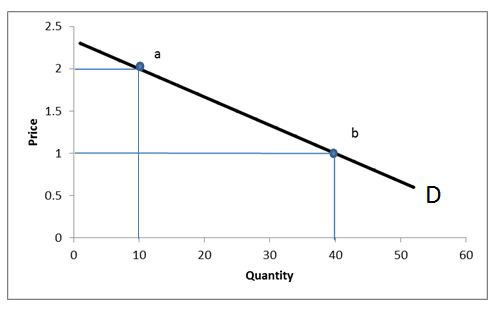The formula for calculating price elasticity of demand using the midpoint formula isNote that from point a and point b, we have everything we need to substitute into the expression. Substituting, we haveA common thing that is done for price elasticity of demand is that the negative sign is dropped, because the number will always be negative and comparing negative numbers may cause unnecessary confusion. Since 1.8 is greater than 1, the demand for the good is elastic. In the same way that we calculated price elasticity above, we use the two new points c and d, which are (10,4) and (20,1), respectively, to find the price elasticity of demand.Once again, ignoring the negative sign, we see that 0.556 is between 0 anD1, so the demand for the good is inelastic. As we have calculated price elasticity of demand above, we use the two new points e and f, which are (10,3) and (30,1), respectively, to find the price elasticity of demand.Ignoring the negative sign, we have that the price elasticity of demand is exactly 1, and so the demand for this good is unit elastic.

Elasticity of demand measures the responsiveness of change in demand due to change in price. Price elasticity of demand measures the responsiveness of change in quantity demanded due to change in price. Elasticity varies along the downward sloping demand curve. The upper segment of the demand curve has elastic demand, the lower portion has inelastic demand and the elasticity is equal to unity at the center of the demand curve. Below is the formula used to calculate mid-point price elasticity of demand: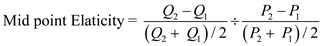Calculate price elasticity of demand between 1 anD2 units:Calculate price elasticity of demand between 1000 anD2000 units:From the above it can be observed that the price elasticity of demand remains same irrespective of the quantity demandeD1 unit anD2 units or 1000 units anD2000 units.

The elasticity of demand: It is the ratio of percentage change in quantity demand due to the percentage change in price. It can be elastic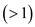, inelastic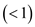or unitary elastic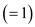. The following figure shows the shift of elastic demand curve: Graph 1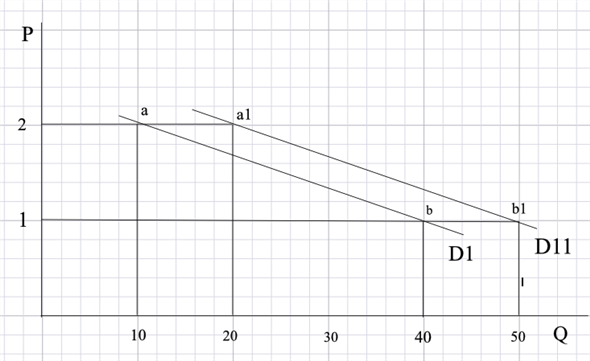With the increase in the demand by 10 units, the new demand curve shifts to D11 by 10 units at the price of \$1 and \$2. The new demand points are a1 (\$2, 20) and b1 (\$1, 50). The following figure shows the shift of the inelastic demand curve: Graph 2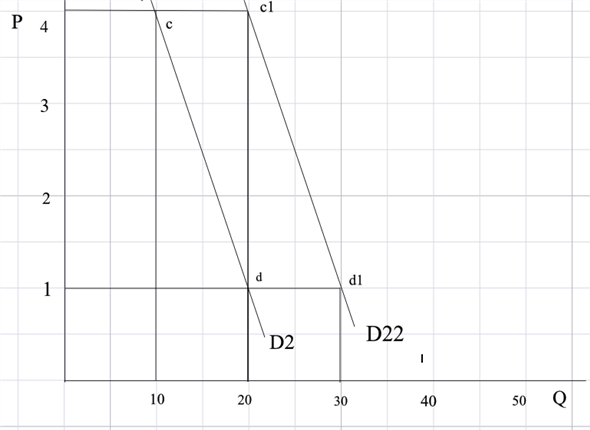With the increase in the demand by 10 units, the new demand curve shifts to D22 by 10 units at the price of \$1 and \$4. The new demand points are c1 (\$4, 20) and d1 (\$1, 30). The following figure shows the shift of unit-elastic demand curve: Graph 3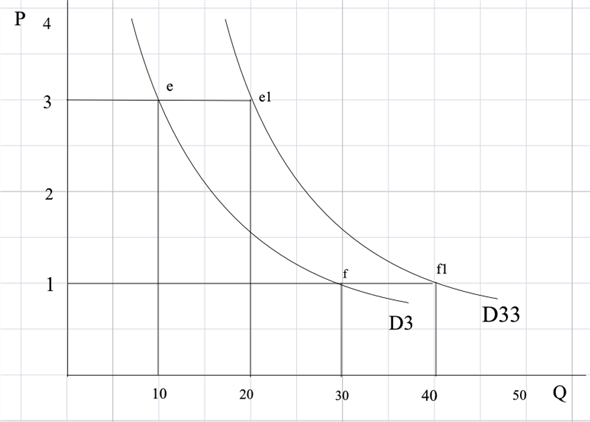With the increase in the demand by 10 units, the new demand curve shifts to D3 by 10 units at the price of \$1 and \$3. The new demand points are e1 (\$3, 20) and f1 (\$1, 30). The formula for calculating the price elasticity of demand using the midpoint formula is shown below:Elasticity for graph 1 Using new demand points of graph 1 [a1 (\$2, 20), b1 (\$1, 50)], calculate the elasticity of demand using mid-point formula as follows:A common thing that is done for price elasticity of demand is that the negative sign is dropped. This is because the number will always be negative and comparing negative numbers may cause unnecessary confusion. Since 1.286 is greater than 1, the demand for the good is elastic. Using the original points (10, \$2) and (40, \$1), we haveOnce again, the negative sign is dropped. The demand for this good is still elastic since 1.8 is greater than 1. When the demand increased, the price elasticity of demand fell from 1.8 to 1.286. Elasticity for graph 2 Using new demand points of graph 2 [c1 (\$4, 20), d1 (\$1, 30)], calculate the elasticity of demand using mid-point formula as follows:Once again, ignoring the negative sign, the elasticity of demand is 0.333 lies between 0 anD1, so the demand for the good is inelastic. Using the original points (10, \$4) and (20, \$1), find the price elasticity of demand as follows:Ignoring the negative sign, it is observed that the price elasticity of demand using the original two points is 0.556. Once again, when demand increased, the price elasticity of demand fell (from 0.556 to 0.333). Elasticity for graph 3 Using new demand points of graph 2 [e1 (\$3, 20) and f1 (\$1, 40)], calculate the elasticity of demand using mid-point formula as follows:Ignoring the negative sign, it is observed that the price elasticity of demand is 0.667, which means the demand for the good is inelastic because it is between 0 anD1. Using the original two points, (10,3) and (30,1), find the price elasticity of demand as follows:Ignoring the negative sign, it is observed that the price elasticity of demand is exactly 1, and so the demand for this good is unit elastic. Once again, the increase in demand caused price elasticity of demand to go down (here, e d falls from 1 to 0.667). The change in the elasticity is caused by the change in the percentage change in quantity demanded. Note that while the change in demand is the same (since demand increases by 10 units, the change in demand will be exactly as it was before), the percentage change in demand will be different. To give an example, suppose there are two points (10, \$1) and (11, \$2). Going from a quantity of 10 to a quantity of 11 here is a change of one unit, but the percentage change from 10 to 11 is a 10% increase. Now, consider the two points (100, \$1) and (101, \$2). Once again, there is only one unit change, but percentage-wise, it is now a 1% increase. This is essentially what was happening when 10 is added to the demand - the numbers for quantity demanded are bigger so the percentage change became smaller, and elasticity fell.